Want to share your content on R-bloggers? click here if you have a blog, or here if you don't.

Last week, I presented an analysis on the longitudinal development of
intelligibility in children with cerebral palsy—that is, how well do strangers
understand these children’s speech from 2 to 8 years old. My analysis used a
Bayesian nonlinear mixed effects Beta regression model. If some models are livestock and some are
pets
, this
model is my dearest pet. I first started developing it a year ago, and it took
weeks of learning and problem-solving to get the first version working
correctly. I was excited to present results from it.

But here’s the thing. I couldn’t just formally describe my model. Not for this
audience. My talk was at the annual conference of the American
Speech-Language-Hearing Association: The main professional gathering for
speech-language pathologists, audiologists, and researchers in communication
sciences and disorders. The audience here is rightly more concerned with
clinical or research matters than the nuts and bolts of my model.

Still, I wanted to convey the main ideas behind the model without dumbing things down. I got to work
making lots of educational diagrams. Among them was the annotated logistic curve
from my earlier post and the following
figure, used to illustrate information borrowing (or partial pooling) in mixed
effects models:

I am pleased with this figure. I originally tried to convey the idea as an
interactive process: Individual-level data inform the population model and those
feed back into the individual estimates. I had only two sets of plots with
labeled paths running back and forth between them; it wasn’t pretty. This plot’s
“feed-forward” approach simplified things a great deal. My only concern, in
hindsight, is that I should have oriented things to run left-to-right instead of
top-to-bottom so I could have filled a 16:9 widescreen slide better. (But this
vertical version probably looks great on your phone or tablet right now, so
whatever!)

In this post, I walk through how to produce a plot like this one from scratch. I
can’t share the original model or the clinical data here, so I will use the
`sleepstudy` data from lme4, as in my partial pooling
tutorial
.

First, let’s set up our data.

``````library(tidyverse)
library(brms)
library(tidybayes)
library(cowplot)

# Convert to tibble for better printing. Convert factors to strings
sleepstudy <- lme4::sleepstudy %>%
as_tibble() %>%
mutate(Subject = as.character(Subject))

# Add two fake participants, as in the earlier partial pooling post
df_sleep <- bind_rows(
sleepstudy,
tibble(Reaction = c(286, 288), Days = 0:1, Subject = "374"),
tibble(Reaction = 245, Days = 0, Subject = "373"))

df_sleep
#> # A tibble: 183 x 3
#>    Reaction  Days Subject
#>
#>  1     250.     0 308
#>  2     259.     1 308
#>  3     251.     2 308
#>  4     321.     3 308
#>  5     357.     4 308
#>  6     415.     5 308
#>  7     382.     6 308
#>  8     290.     7 308
#>  9     431.     8 308
#> 10     466.     9 308
#> # ... with 173 more rows

# Select four participants to highlight
df_demo <- df_sleep %>%
filter(Subject %in% c("335", "333", "350", "374"))
``````

We fit a mixed model with default priors and a random-number seed for
reproducibility.

``````b <- brm(
Reaction ~ Days + (Days | Subject),
data = df_sleep,
seed = 20191125
)
b
``````
``````#>  Family: gaussian
#>   Links: mu = identity; sigma = identity
#> Formula: Reaction ~ Days + (Days | Subject)
#>    Data: df_sleep (Number of observations: 183)
#> Samples: 4 chains, each with iter = 2000; warmup = 1000; thin = 1;
#>          total post-warmup samples = 4000
#>
#> Group-Level Effects:
#> ~Subject (Number of levels: 20)
#>                     Estimate Est.Error l-95% CI u-95% CI Rhat Bulk_ESS Tail_ESS
#> sd(Intercept)          25.84      6.26    15.17    39.80 1.00     1823     2642
#> sd(Days)                6.55      1.50     4.19     9.99 1.00     1607     1965
#> cor(Intercept,Days)     0.09      0.29    -0.45     0.66 1.00      904     1618
#>
#> Population-Level Effects:
#>           Estimate Est.Error l-95% CI u-95% CI Rhat Bulk_ESS Tail_ESS
#> Intercept   252.49      6.77   239.10   266.00 1.00     2115     2502
#> Days         10.49      1.71     7.26    14.07 1.00     1411     2073
#>
#> Family Specific Parameters:
#>       Estimate Est.Error l-95% CI u-95% CI Rhat Bulk_ESS Tail_ESS
#> sigma    25.81      1.55    23.01    29.07 1.00     3271     2891
#>
#> Samples were drawn using sampling(NUTS). For each parameter, Eff.Sample
#> is a crude measure of effective sample size, and Rhat is the potential
#> scale reduction factor on split chains (at convergence, Rhat = 1).
``````

## Top row: connect the dots

Let’s create the top row. The core of the plot is straightforward: We
draw lines, draw points, facet by `Subject`, and set a reasonable y-axis range for
the plot (controlling the coordinates via `coord_cartesian()`).

``````col_data <- "#2F2E33"

p_first_pass <- ggplot(df_demo) +
aes(x = Days, y = Reaction) +
geom_line(aes(group = Subject), color = col_data) +
geom_point(color = col_data, size = 2) +
facet_wrap("Subject", nrow = 1) +
coord_cartesian(ylim = c(200, 500)) +
ggtitle("Each participant contributes data") +
theme_grey(base_size = 14)

p_first_pass
``````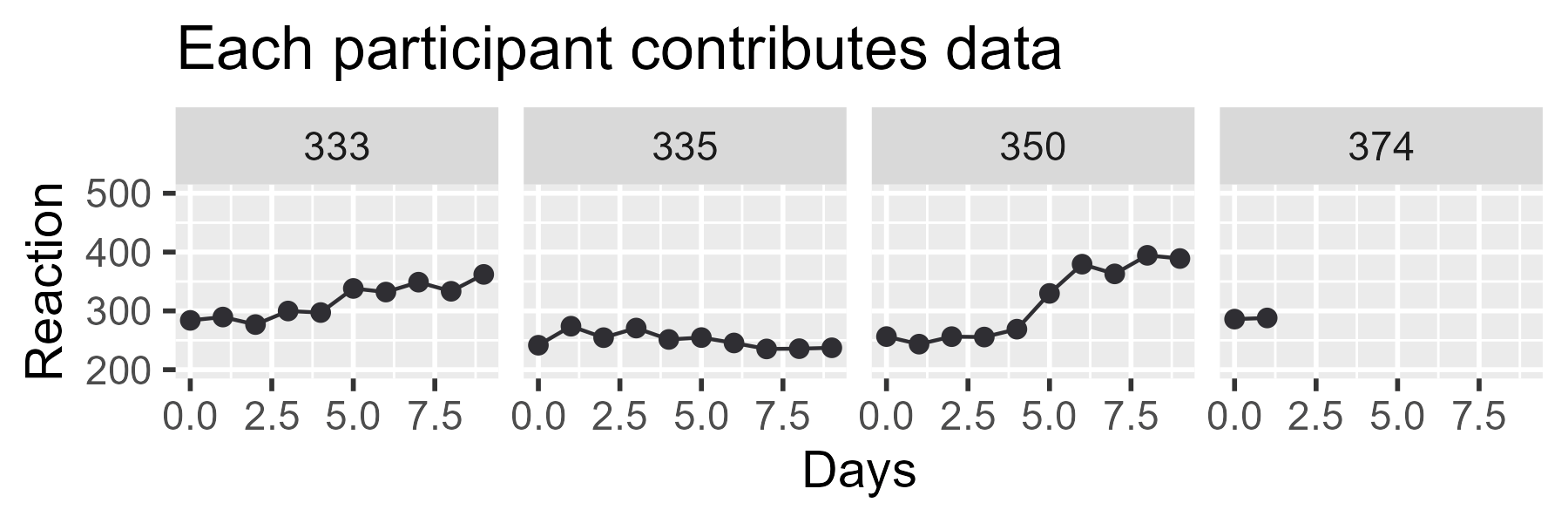First, let’s make one somewhat obscure tweak. Currently, the left edge of the
title is aligned with with plotting panel—that is, the window where the data
are drawn. But in our final ensemble, we are going to have three plots and the left
edge of the panel will not be in the the same location across all three plots.
We want our titles to be aligned with each other from plot to plot, so we tell
ggplot2 to position the plot title using the left edge of the `"plot"`, as opposed
to the `"panel"`.

``````p_first_pass_tweak <- p_first_pass +
theme(plot.title.position = "plot")
p_first_pass_tweak
``````⚠️ Note that the `plot.title.position` `theme()` option is
only available in the development version of ggplot2 as of
November 2019.

For the diagram, we have to remove the facet labels (“strips”),
axis titles, axis text, and axis ticks (those little lines that stick out of the
plot). We also clean up the gridlines for the x axis. The x unit is whole
number `Days`, so putting a line (“break”) at 2.5 is not meaningful.

``````p_second_pass <- p_first_pass_tweak +
scale_x_continuous(breaks = seq(0, 9, by = 2)) +
labs(x = NULL, y = NULL) +
theme(
# Removing things from `theme()` is accomplished by setting them
# `element_blank()`
strip.background = element_blank(),
strip.text = element_blank(),
axis.text = element_blank(),
axis.ticks = element_blank(),
panel.grid.minor = element_blank()
)

p_second_pass
``````Finally, let’s add the “(others)” label. Here, I use the `tag` label
as a sneaky way to add an annotation. Normally, tags are meant to label
individual plots in an ensemble display of multiple plots:

``````ggplot() + labs(title = "A tagged plot", tag = "A. ")
``````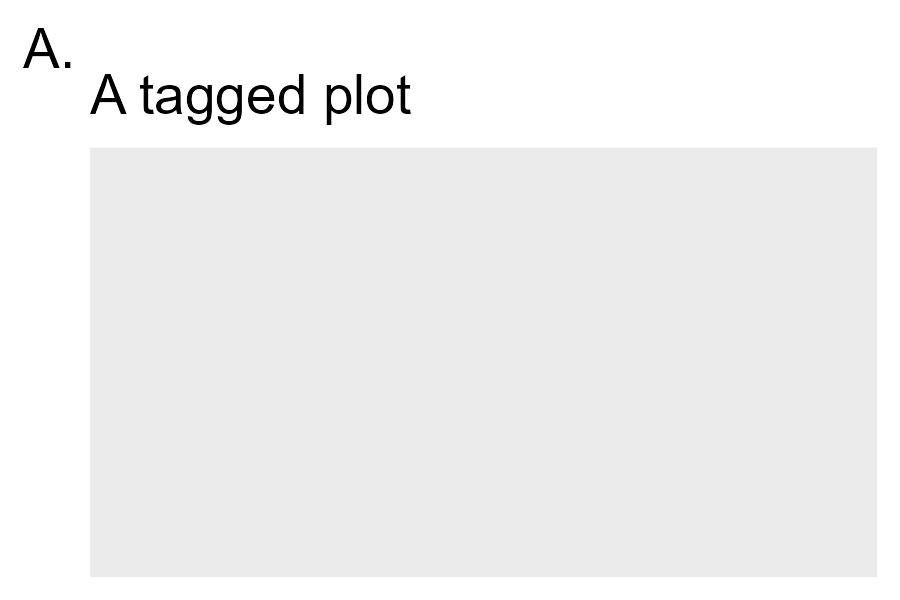But we will use that text feature to place some text to the right side of the
plot. Sometimes, there’s a correct way and there’s the way that gives you the
image you can paste into your slides, and this tag trick is one of two shortcuts
I used in my diagram.

``````tag_others <- "   (others)   "

p_second_pass +
labs(tag = tag_others) +
theme(
plot.tag.position = "right",
plot.tag = element_text(size = rel(.9))
)
``````I have discovered that incrementally and didactically building up my plots over
multiple code chunks creates a hassle for Future Me when copypasting plotting
code, so the final, complete code is below.

``````col_data <- "#2F2E33"
tag_others <- "   (others)   "

p_top <- ggplot(df_demo) +
aes(x = Days, y = Reaction) +
geom_line(aes(group = Subject), color = col_data) +
geom_point(color = col_data, size = 2) +
facet_wrap("Subject", nrow = 1) +
scale_x_continuous(breaks = seq(0, 9, by = 2)) +
coord_cartesian(ylim = c(200, 500)) +
ggtitle("Each participant contributes data") +
labs(x = NULL, y = NULL, tag = tag_others) +
theme_grey(base_size = 14) +
theme(
strip.background = element_blank(),
strip.text = element_blank(),
axis.text = element_blank(),
axis.ticks = element_blank(),
panel.grid.minor = element_blank(),
plot.tag.position = "right",
plot.tag = element_text(size = rel(.9)),
plot.title.position = "plot"
)
``````

## Bottom row: Beams of light

Let’s skip to the bottom row because it uses the same coordinates, points and
theming as the top row. The plot visualizes the posterior fits (the estimated
mean) as a median and a 95% interval.
tidybayes makes the process
straightforward with `add_fitted_draws()` to add model fits onto a dataframe and with
`stat_lineribbon()` to plot a line and ribbon summary of a posterior
distribution.

``````df_demo_fitted <- df_demo %>%
# Create a dataframe with all possible combination of Subject and Days
tidyr::expand(
Subject,
Days = seq(min(Days), max(Days), by = 1)
) %>%
# Get the posterior predictions
``````

Now we have 4,000 posterior samples for the fitted `Reaction` for each
`Subject` for each day.

``````df_demo_fitted
#> # A tibble: 160,000 x 7
#> # Groups:   Subject, Days, .row 
#>    Subject  Days  .row .chain .iteration .draw .value
#>
#>  1 333         0     1     NA         NA     1   272.
#>  2 333         0     1     NA         NA     2   264.
#>  3 333         0     1     NA         NA     3   266.
#>  4 333         0     1     NA         NA     4   286.
#>  5 333         0     1     NA         NA     5   269.
#>  6 333         0     1     NA         NA     6   270.
#>  7 333         0     1     NA         NA     7   265.
#>  8 333         0     1     NA         NA     8   283.
#>  9 333         0     1     NA         NA     9   246.
#> 10 333         0     1     NA         NA    10   269.
#> # ... with 159,990 more rows
``````

Given these posterior fits, we call `stat_lineribbon()` to get the median
and 95% intervals. The plotting code is otherwise the same as the last one,
except for a different title and two lines that set the fill color and hide the fill legend.

``````col_data <- "#2F2E33"
tag_others <- "   (others)   "

p_bottom <- ggplot(df_demo_fitted) +
aes(x = Days, y = .value) +
# .width is the interval width
stat_lineribbon(alpha = .4, .width = .95) +
geom_point(
aes(y = Reaction),
data = df_demo,
size = 2,
color = col_data
) +
facet_wrap("Subject", nrow = 1) +
# Use the viridis scale on the ribbon fill
scale_color_viridis_d(aesthetics = "fill") +
# No legend
guides(fill = FALSE) +
scale_x_continuous(breaks = seq(0, 9, by = 2)) +
coord_cartesian(ylim = c(200, 500)) +
ggtitle(
"Individual trajectories \"borrow information\" from others"
) +
labs(x = NULL, y = NULL, tag = tag_others) +
theme_grey(base_size = 14) +
theme(
strip.background = element_blank(),
strip.text = element_blank(),
axis.text = element_blank(),
axis.ticks = element_blank(),
panel.grid.minor = element_blank(),
plot.tag.position = "right",
plot.tag = element_text(size = rel(.9)),
plot.title.position = "plot"
)

p_bottom
``````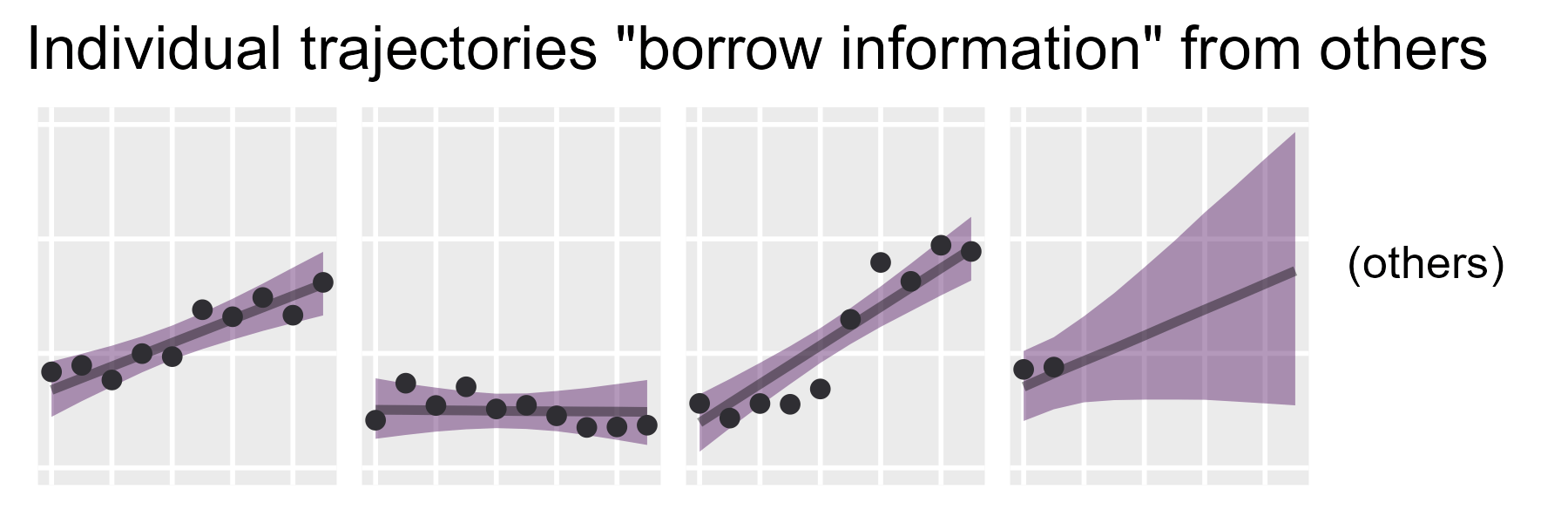## Middle row: Piles of ribbons

For the center population plot, we are going to use posterior predicted means
for a new (as yet unobserved) participant. This type of prediction incorporates
the uncertainty for the population average (i.e., the fixed effects) and the
population variation (i.e., the random effects).

We do this by defining a new participant and obtaining their posterior fitted
values. I like to use `"fake"` as the ID for the hypothetical participant.

``````df_population <- df_sleep %>%
distinct(Days) %>%
mutate(Subject = "fake") %>%
``````

Next, it’s just a matter of using `stat_lineribbon()` again with many, many
`.width` values to recreate that center visualization.

``````ggplot(df_population) +
aes(x = Days, y = .value) +
stat_lineribbon(
.width = c(.1, .25, .5, .6, .7, .8, .9, .95)
) +
scale_x_continuous(
"Days",
breaks = seq(0, 9, by = 2),
minor_breaks = NULL
) +
coord_cartesian(ylim = c(200, 500)) +
scale_y_continuous("Reaction Time") +
scale_color_viridis_d(aesthetics = "fill") +
guides(fill = FALSE) +
ggtitle("Model estimates the population of participants") +
theme_grey(base_size = 14) +
theme(plot.title.position = "plot")
``````Actually, not quite. That median line ruins everything. It needs to go.

I’m too lazy to figure out how to get `stat_lineribbon()` to draw just the
ribbons. Instead, note that colors in ggplot2 can be 8-digit
hex codes, where the last two digits set the transparency for the color.

``````# tidybayes::stat_lin
ggplot() +
geom_vline(xintercept = 1, size = 4, color = "#000000FF") +
geom_vline(xintercept = 2, size = 4, color = "#000000CC") +
geom_vline(xintercept = 3, size = 4, color = "#000000AA") +
geom_vline(xintercept = 4, size = 4, color = "#00000077") +
geom_vline(xintercept = 5, size = 4, color = "#00000044") +
geom_vline(xintercept = 6, size = 4, color = "#00000011") +
ggtitle("Using 8-digit hex colors for transparency values") +
theme(panel.grid = element_blank())
``````This is the other shortcut I used in this diagram: I tell `stat_lineribbon()` to use
some color with `00` for the final 2 digits, so that it draws the median as a
completely transparent line.

``````p_population <- ggplot(df_population) +
aes(x = Days, y = .value) +
stat_lineribbon(
# new part
color = "#11111100",
.width = c(.1, .25, .5, .6, .7, .8, .9, .95)
) +
scale_x_continuous(
"Days",
breaks = seq(0, 9, by = 2),
minor_breaks = NULL
) +
coord_cartesian(ylim = c(200, 500)) +
scale_y_continuous("Reaction Time") +
scale_color_viridis_d(aesthetics = "fill") +
guides(fill = FALSE) +
ggtitle("Model estimates the population of participants") +
theme_grey(base_size = 14) +
theme(plot.title.position = "plot")
p_population
``````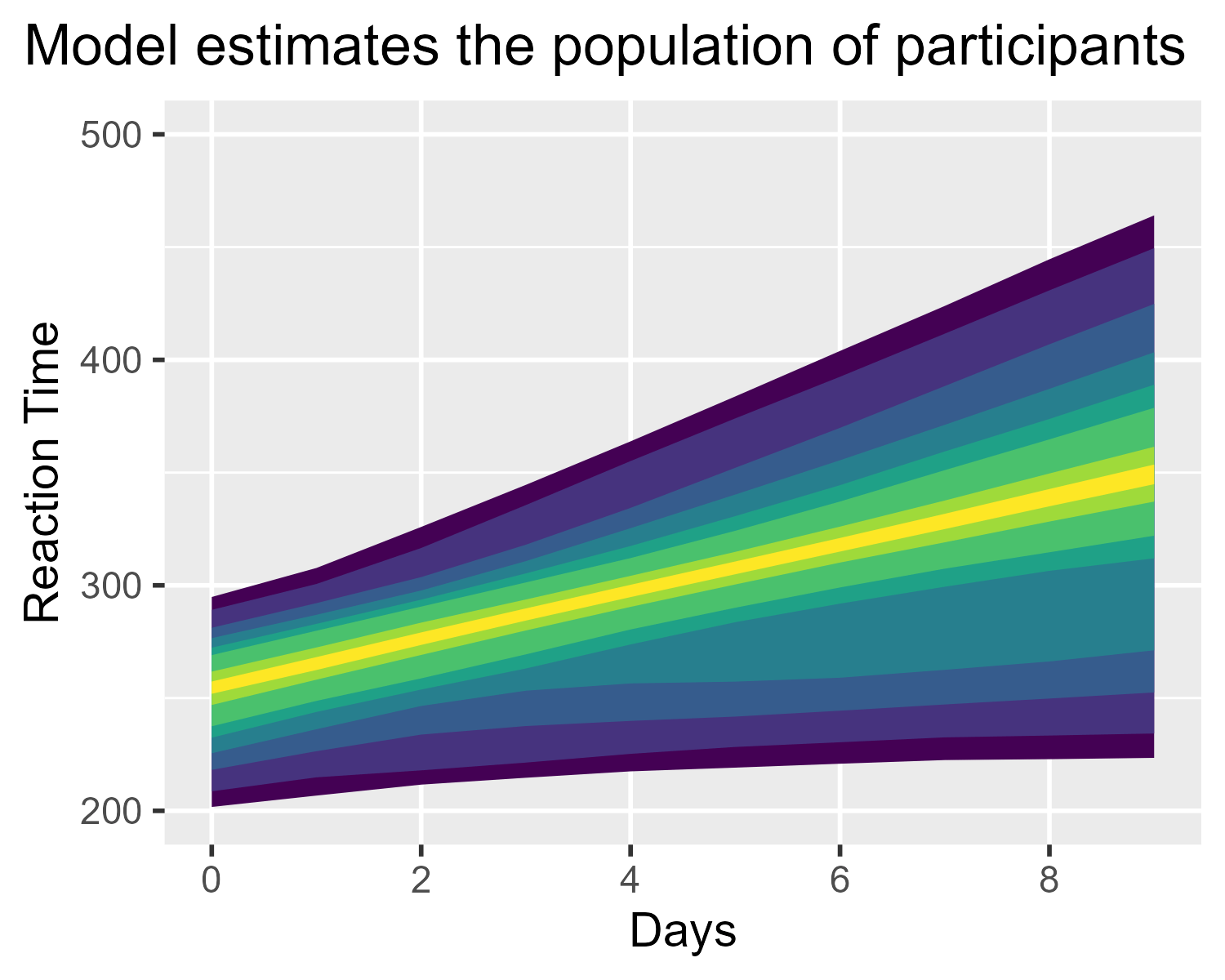## Mooooooo: cowplot time

Finally, we use `plot_grid()` from cowplot1 to put things together.
First, I don’t want the middle population plot to be as wide as the top and
bottom rows, so I first create a `plot_grid()` containing the center plot and an
empty `NULL` spacer to its right. (I recommend this
vignette
for learning how
to use `plot_grid()`.)

``````p_middle <- plot_grid(p_population, NULL, nrow = 1, rel_widths = c(3, .5))
``````

Now, we just stack three on top of each other in to column:

``````plot_grid(
p_top,
p_middle,
p_bottom,
ncol = 1,
rel_heights = c(1, 2, 1)
)
``````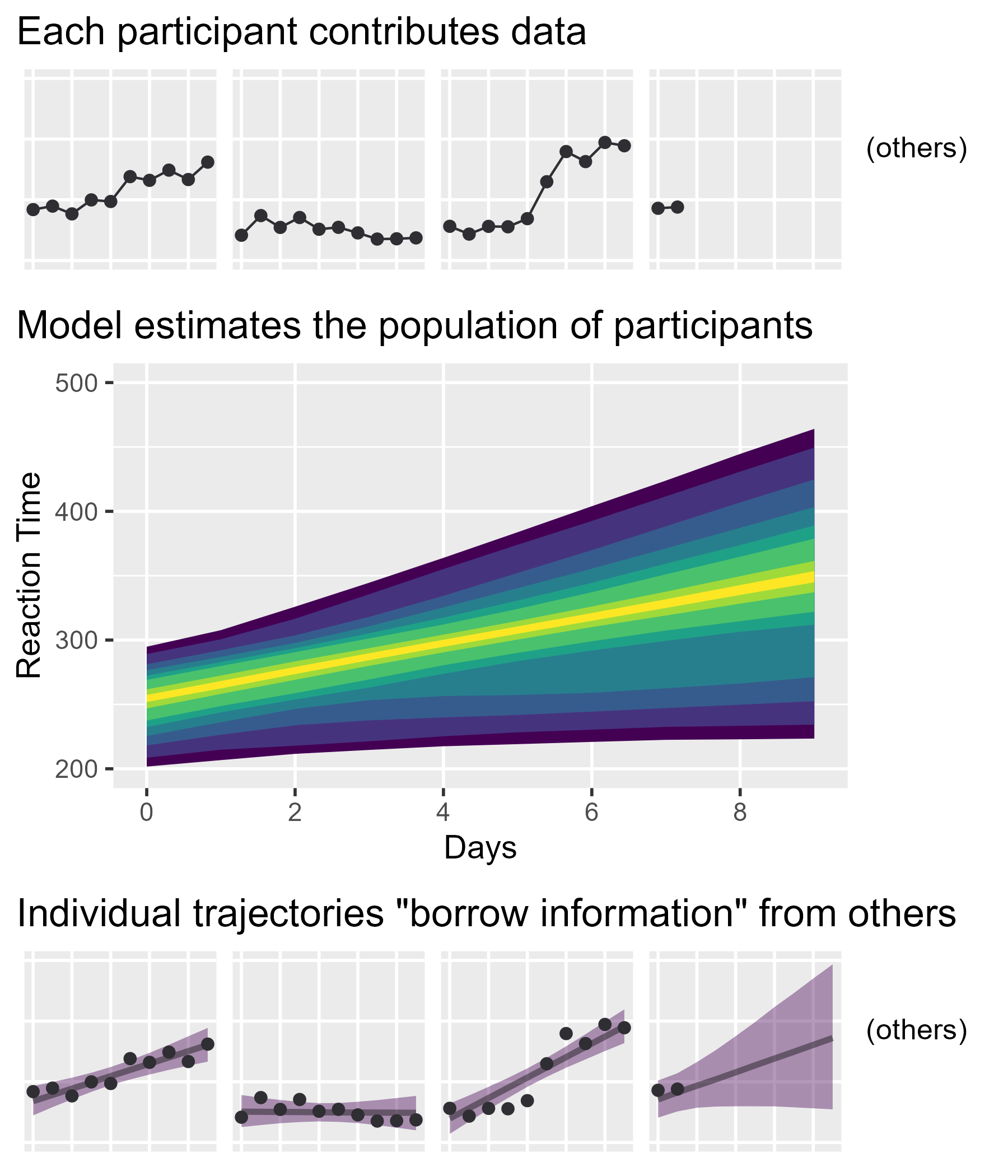That’s it! It’s amazing how much work tidybayes and cowplot save us in making
these plots. In fact, without being aware of them or their capabilities, I might
have been discouraged from even trying to create this diagram.

1. As I’ve mentioned elsewhere on this site, I grew up on a dairy farm 🐮, so I always read cowplot with an agricultural reading: These are plots of space that are being fenced off and gridded together, like one might see in an aerial shot of farmland. But the package author is Claus O. Wilke, so it’s probably just C.O.W.’s plotting package. Probably. I’ve never asked and don’t intend to. I don’t want my pastoral notions dispelled.